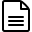# What is the K-Factor of the Floscan Fuel Flow Sensor?

Each Floscan model 201A-6, 201B-6 or 201C-6 is shipped with a tag attached to it. This tag has a number that looks something like this:

16-3200

What this means is that the sensor was calibrated at 16 GPH to have a K-factor of 32,000 (you have to multiply the last 4 digits by 10 for K-factor).

However, this is a Pulse Per Gallon K-factor and the MGL Avionics systems run on a Pulse Per Liter internal calculation. Therefore, you need to take the Floscan K-factor, and divide it by 3.785

So,

3200 x 10 =
32,000
/ 3.785 =

K-factor of 8,454Note: this is just in this example - you need to use the tag that came with the sensor. If you no longer have the tag, start with a K-factor of approx 9,100 and then check it over time and recalibrate.